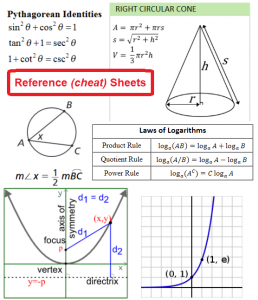# How Should We React to a Formula Sheet that Appears Overwhelming at First Glance?

A formula/reference sheet (for geometry, algebra, or trigonometry, for example) can be overwhelming.

We can turn it into a useful tool by doing the following:1. Identify and focus on the big key ideas of the sheet, with which we need to become fluent (know deeply and use effectively).
2. Become familiar with the overall structure and terminology of the formula sheet.
3. Identify the key connecting themes.  Understand how various aspects of the sheet are related.
4. Understand the ideas conceptually.  The brain remembers meaning better than abstract symbols.
5. Identify the technical details which will be handy to look up, when needed, but which we will not memorize.

Formula Sheets

Here are some formula sheets (aka reference sheets or cheat sheets) I like.